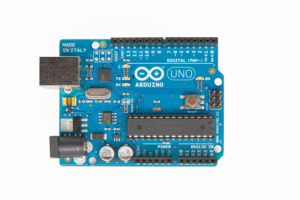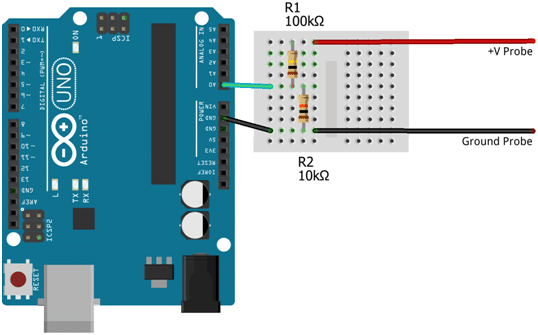Multimeters and voltmeters are essential pieces of equipment when working on any kind of electronics circuit or Arduino project. A voltmeter measures the voltage between two points in a circuit and, with digital electronics, this is an analog range from zero to the level of the power supply (Vcc). Digital voltmeters work by converting the analog value to a digital value using an analog to digital converter (ADC). A typical Arduino has several of these converters on the board, and in this tutorial you will see how to read voltages from the Arduino’s analog inputs and build a voltmeter that measures direct current (DC) voltages.

The circuit and information presented below assume a basic knowledge of digital electronics and circuits, and how to use an Arduino. Learning the Arduino is a step-by-step process, covering both programming in C and working with electronics circuits.

To complete the tutorial, you will need:

• The Arduino IDE (integrated development environment).
• One 100Ko resistor.
• One 10Ko resistor.
• Four wires, in at least two different colors (red and black are recommended).
• A breadboard (or suitable stripboard and soldering equipment).

In preparation, you should solder crocodile clips to two differently-colored wires, as this will make it easier to attach them to components when measuring voltages.

Working with electricity, even at low voltages, can be dangerous. There a risk of damage to equipment and yourself – follow the connection diagrams and instructions carefully, and always seek advice from a qualified and experienced adult if you are unsure.

## The Arduino Sketch

To eliminate the possibility that the Arduino will run a previous sketch and operate in an unknown way, you can program the sketch first.

To create the voltmeter sketch:

1. Open the Arduino IDE.
2. Paste in the following code:
```/*
*
* Udemy.com
* Building an Arduino DC Voltmeter
*
*/
float vPow = 4.7;
float r1 = 100000;
float r2 = 10000;
void setup() {
Serial.begin(9600);

// Send ANSI terminal codes
Serial.print("\x1B");
Serial.print("[2J");
Serial.print("\x1B");
Serial.println("[H");
// End ANSI terminal codes

Serial.println("--------------------");
Serial.println("DC VOLTMETER");
Serial.print("Maximum Voltage: ");
Serial.print((int)(vPow / (r2 / (r1 + r2))));
Serial.println("V");
Serial.println("--------------------");
Serial.println("");

delay(2000);
}
void loop() {
float v = (analogRead(0) * vPow) / 1024.0;
float v2 = v / (r2 / (r1 + r2));

// Send ANSI terminal codes
Serial.print("\x1B");
Serial.print("[1A");
// End ANSI terminal codes

Serial.println(v2);
}```

And then save the sketch:

1. On the File menu, click Save As…

This sketch begins by initializing the serial port and declaring a few variables:

vPow – When powered over a USB cable, it is common for the Arduino’s 5V power supply to be a little less than that ideal.

r1 – the value (in ohms) of the first resistor in the circuit.

r2 – the value (in ohms) of the second resistor in the circuit.

The sketch then sends some basic information to the terminal, and it displays the maximum voltage than can safely be measured by the current circuit.

The Serial Port Monitor in the IDE can be used to view the messages sent by this sketch. However, this is not a particularly advanced monitor, and cannot display the ANSI terminal sequences that are used to provide a friendlier display. Better results can be had by using a terminal package such as Hyperterminal, RealTerm or Putty.

The serial port you need to connect to can be found from the Arduino IDE:

• On the Tools menu, click Serial Port and look for the item that is ticked.

The other setting you should use are:

Display: ANSI

Speed: 9600

Parity: None

Data Bits: 8

Stop Bits: 1

Hardware Flow Control: None

Software Flow Control: None

## Building the Circuit

Disconnect the Arduino from your computer before building this circuit!

The circuit can be constructed on a breadboard:The analog inputs of an Arduino can measure up to 5V (when using the built-in analog reference voltage). Even when only connecting to a 5V circuit, you should use the resistors to help protect the Arduino from short-circuits or unexpected voltage surges.

Those two resistors form a potential divider that is used to lower the voltage being measured to a level that the Arduino can read. This actually extends the range that can be used. For example, if resistors are used to halve the input voltage then the Arduino can effectively read up to 10V (since 10V will be read as 5V, 5V will be read as 2.5V…). This comes at the expensive of accuracy – the ADCs in the Arduino can read up to 1024 different levels between 0V and 5V. By expanding the range to 10V, those 1024 levels are spread across a wider range and are therefore less able to detect small changes.

You can increase the resistance value of R2, then the maximum voltage that can be read will be decreased; giving a slightly more accurate reading. With R1 at 100Ko and R2 at 10Ko, the input voltage is reduced by a factor of around 11 – allowing the voltmeter to read from 0V–55V.

The formula for calculating values in a potential divider is:

`Vout = (R2 / (R1 + R2)) * Vin`

If the divider for the Arduino voltmeter is functioning correctly then Vout will be a maximum of 5V, and so you can calculate the maximum input voltage to the circuit:

`Vmax = 5.0 / (R2 / (R1 + R2))`

You can see a variation of this expression used in the setup() routine of the sketch.

Note: If you use different resistors from the ones suggested here, you must be remember to adjust the values of r1 and r2 in the sketch.

When measuring the voltage in the loop() routine, analogRead(0) is used to read the level from analog input 0. The returned value is an integer in the range 0 through 1023, so it must first be adjusted to a range 0 through 5. This is done by multiplying it by the power supply level, and then dividing by 1024.

To transform the 0V–5V value into a reading that reflects the range of values that can be measured by the circuit, the resistors must be taken into account in the same way as was done to calculate the maximum voltage the circuit could measure:

`v2 = v / (r2 / (r1 + r2))`

With all the calculations completed, the value now represents the actual voltage measured by the circuit, and is sent to the display.

## Enhancing the Voltmeter

The voltmeter presented here is extremely basic and there is considerable room for enhancements, such as using switches to allow the user to choose from multiple potential dividers; adding an LCD display or a front-end in Processing; or building the meter using a minimal AVR circuit instead of a full Arduino board. Arduino Step-by-Step offers a more comprehensive look at the projects that can be created, and how to work with different types of components.

Page Last Updated: May 2014

### Top courses in Arduino

Arduino Step by Step Getting Serious
Dr. Peter Dalmaris
4.6 (1,479)
Arduino Step by Step: Getting Started
Dr. Peter Dalmaris
4.6 (7,458)
Bestseller
Learn to Design Your Own Boards
Robert Feranec
4.6 (2,677)
Bestseller
Arduino For Beginners - 2023 Complete Course
Edouard Renard
4.6 (1,381)
Bestseller
The Complete Beginners Guide to The Arduino - 2023
Mike McRoberts
4.8 (322)
Highest Rated
Basic electronics for Arduino Makers
Dr. Peter Dalmaris
4.7 (1,934)
Arduino Programming and Hardware Fundamentals with Hackster
Shawn Hymel, ~ Hackster.io ~
4.5 (4,463)
Programming the Arduino - Getting Started
Edouard Renard
4.5 (1,096)
Highest Rated